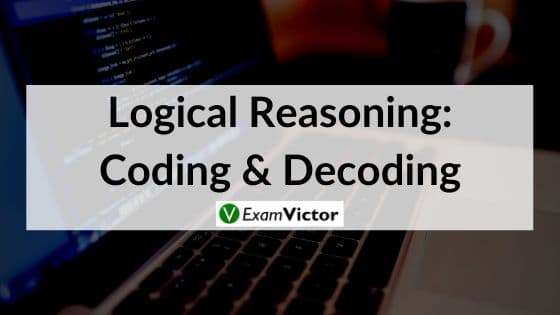# Logical Reasoning: Coding and DecodingLast Updated on Nov 2, 2020

Coding and Decoding is a part of the Reasoning Ability section. You can either score brilliantly or waste a lot of time with no satisfactory output. The more prepared you are, the better is your score.

This article will take you through Coding and Decoding concepts and smart ways to solve questions based on Coding and Decoding. Let’s first understand what coding and decoding is? What types of questions are asked from this topic?

#### What is Coding and Decoding?

• Coding is a way of transmitting data between the sender and the receiver in an encrypted manner i.e. without any other person knowing about it.
• Before transmission, the data is encoded and when it is received, it is decoded in order to obtain the original data.
• This prevents the data from getting corrupt and leaked.

#### Types of coding:

1. Letter Coding
2. Substitution coding
3. Mixed letter coding
4. Mixed number coding
##### Letter Coding

Example: If the code of the word TEACHER is XHDIKHL, what will be the code of HEAT?

Solution: Observe the question closely and you should be able to conclude that there is no relation between the letters of the word TEACHER and the letters of the given code, but the letters of the word HEAT are already contained in the parent word TEACHER. So, picking the corresponding code from the parent word, the code for HEAT will be KHDX.

##### Substitution:

In such questions, names/words are substituted with different names/words. We have to carefully trace the substitution to arrive at the correct answer.

For instance: If ‘Parrot’ is known as ‘Peacock’, ‘Peacock’ is known as ‘Swallow’, ‘Swallow’ is known as ‘Pigeon’, ‘Pigeon’ is known as ‘Sparrow’, what would be the name of the national bird?

Explanation: We know ‘peacock’ is the national bird. But in the given question, ‘Peacock’ is coded as ‘Swallow’. So ‘Swallow’ is the correct answer.

##### Mixed letter coding

In Mixed letter coding type of questions, three or four complete messages are given in the coded language and the code for a particular word is asked. To analyse such codes, any two messages bearing a common word are picked up. The common code word will mean that word. Proceeding smilarly by picking up all possible combinations of two, the entire message can be analysed.

For Example:

In a certain code language,

1. ‘pod na joc’ means ‘very bright boy’;
2. ‘tam nu pod’ means ‘the boy comes’;
3. ‘nu per ton’ means ‘keep the doll’;
4. ‘joc ton su’ means ‘very good doll’.

Which of the following means ‘bright’ in the same code language?
1. joc   2. pod   3. ton   4. na    5.None of these

Ans: D

Explanation:

In statements (a) and (b), the common code word is ‘pod’ and the common word is ‘boy’.
So, ‘pod’ stands for ‘boy’.
In statements (a) and (d), the common code word is ‘joc’ and the common word is ‘very’.
So, ‘joc’ stands for ‘very’.
So, in (a) ‘na’ stands for ‘bright’.

##### Mixed Number Coding

In this type of questions, numbers are given corresponding to phrases. Following the same rule as in the above case, we can easily find out the answer. Selecting two phrases at a time, you have to find out the number code for each word and then arrive at the correct answer.

For Example:

In a certain code,

‘786’ means ‘study very hard’,
‘958’ means ‘hard work pays’
‘645’ means ‘study and work’.

Which of the following is the code for ‘very’?
1. 7   2. 5   3. 8  4.6  5. Either 6 or 7

Ans: 1

Explanation:
In the first and second statements, the common word is ‘hard’ and the common code digit is ’8’. So, ‘8’ means ‘hard’.
In the first and third statements, the common word is ‘study’ and the common code digit is ‘6’. So, ’6’ means ‘study’.
Thus, in the first statement ‘7’ means ‘very’.

### Summary

In this post, we have provided basics of Coding and Decoding, types with easy examples .